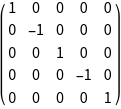GROUP THEORY TUTORIAL

# Angular Momentum Operations

GTPack [1,2] contains various modules to handle angular momentum operators and representations.

•  W. Hergert, R. M. Geilhufe, Group Theory in Solid State Physics and Photonics: Problem Solving with Mathematica, Wiley-VCH, 2018  R. M. Geilhufe, W. Hergert, GTPack: A Mathematica group theory package for applications in solid-state physics and photonics, Frontiers in Physics, 6:86, 2018
 GTJx gives the x component of the total angular momentum operator GTJy gives the y component of the total angular momentum operatorXXXX GTJz gives the z component of the total angular momentum operator

The components of the total angular momentum operator in terms of matrix representations acting on a finite sub space indexed by the total angular momentum quantum number J.

Components of the total angular momentum operator
 In:=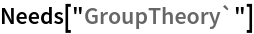In:=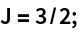In:=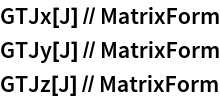Out//MatrixForm=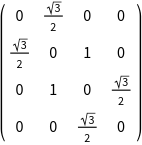Out//MatrixForm=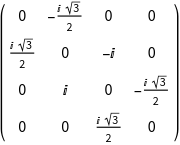Out//MatrixForm=Verify commutation relations.
 In:=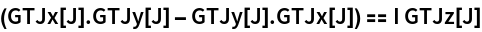Out=In:=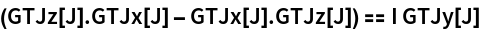Out=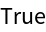In:=Out=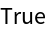GTJplus gives the raising operator GTJminus gives the lowering operator

The raising operator acts as J+|j; m> =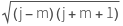|j; m+1>

The lowering operator acts as J-|j; m> =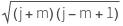|j; m-1>

J+ and J- are related to Jx and Jy:

J+= Jx + i Jy

J-= Jx - i Jy

Matrix representations
 In:=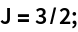In:=Out//MatrixForm=Out//MatrixForm=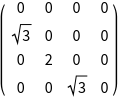Define a state vector
 In:=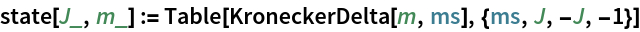Verify action of J+ and normalization.
 In:=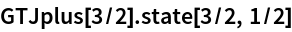Out=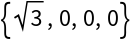In:=Out=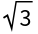Verify action of J+ as a raising operator:
 In:=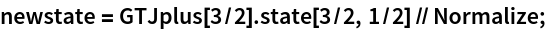In:=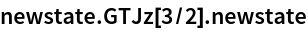Out=Verify action of J- as a lowering operator:
 In:=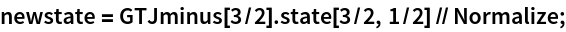In:=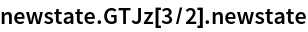Out=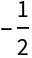Verify commutation relations
 In:=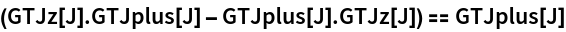Out=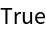In:=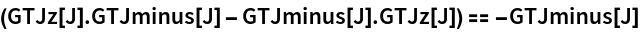Out=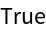In:=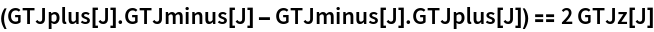Out=Verify relation to Jx and Jy
 In:=Out=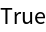In:=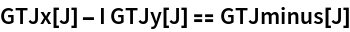Out=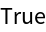GTJTransform applies a symmetry transformation to the basis functions of an irreducible representation of O(3) GTJMatrix gives the representation matrix of a symmetry element for an irreducible representation of O(3)
GTJTransform and GTJMatrix are closely related. While GTJTransform gives the action of a symmetry element on one specific basis function, GTJMatrix gives the transformation matrix of the entire subspace.
 In:=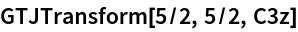Out=In:=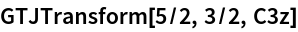Out=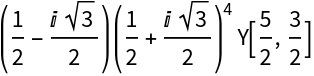In:=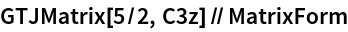Out//MatrixForm=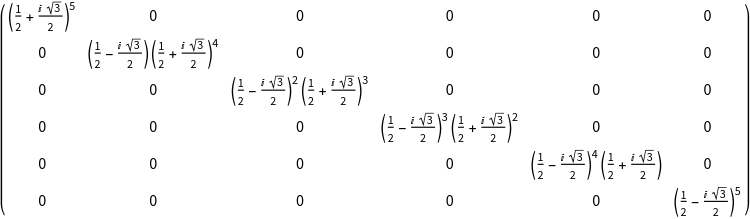GTAngularMomentumRep applies a symmetry transformation to the basis functions of an irreducible representation of O(3) GTAngularMomentumChars gives the representation matrix of a symmetry element for an irreducible representation of O(3)

For the implementation of irreducible representations of O(3), SO(3) and SU(2) we follow .

 Altman, S. L., Rotations, quaternions, and double groups. Chapter 14. Clarendon, 1986

Install the point group Oh (GTInstallGroup)
 In:=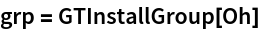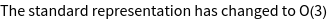Out=Calculate the character Table (GTCharacterTable)
 In:=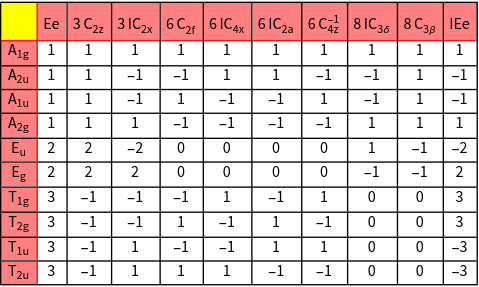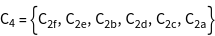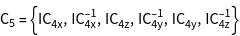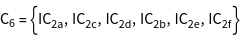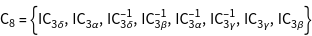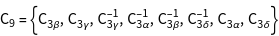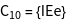Calculate the character system of a single s, p, and d electron
 In:=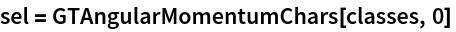Out=In:=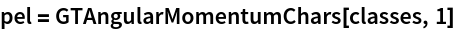Out=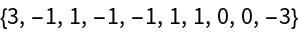In:=Out=Calculate the qualitative splitting in a cubic crystal field
 In:=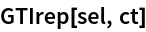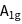Out=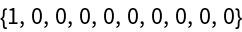In:=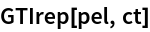Out=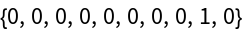In:=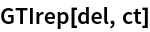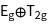Out=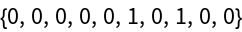Calculate a matrix representation for d-electrons
 In:=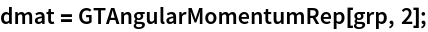In:=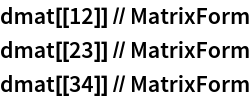Out//MatrixForm=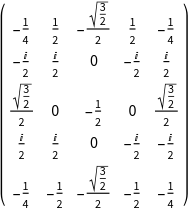Out//MatrixForm=Out//MatrixForm=